##全国校区# 牛逼！用Python为她设计专属签名软件2021-09-28

“不要着急，先去刷刷视频歇一下。”

# 思路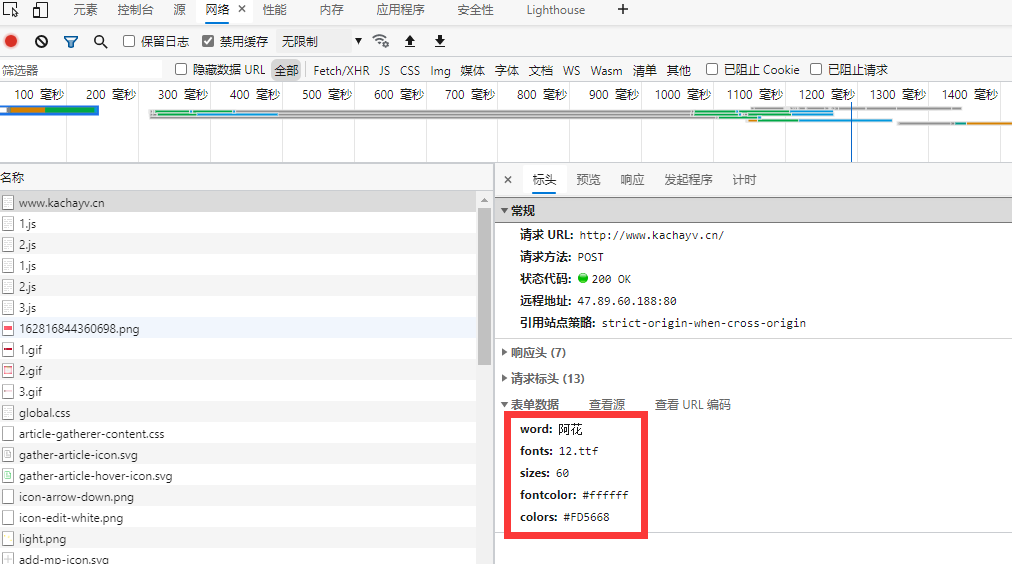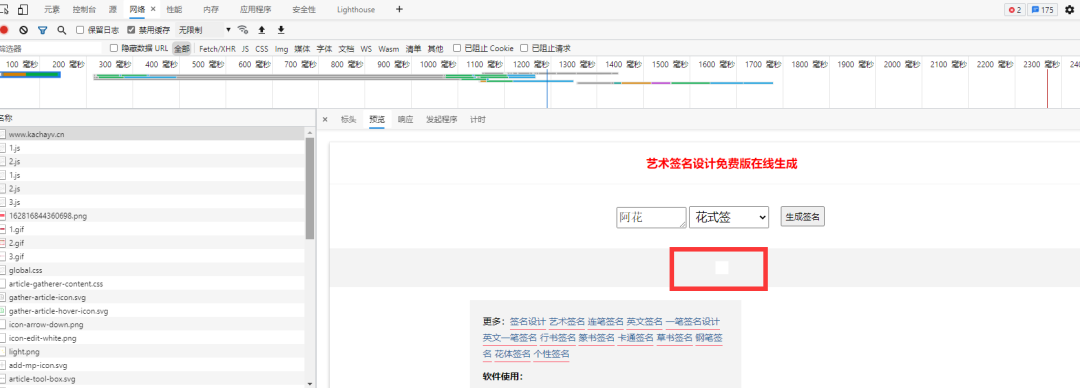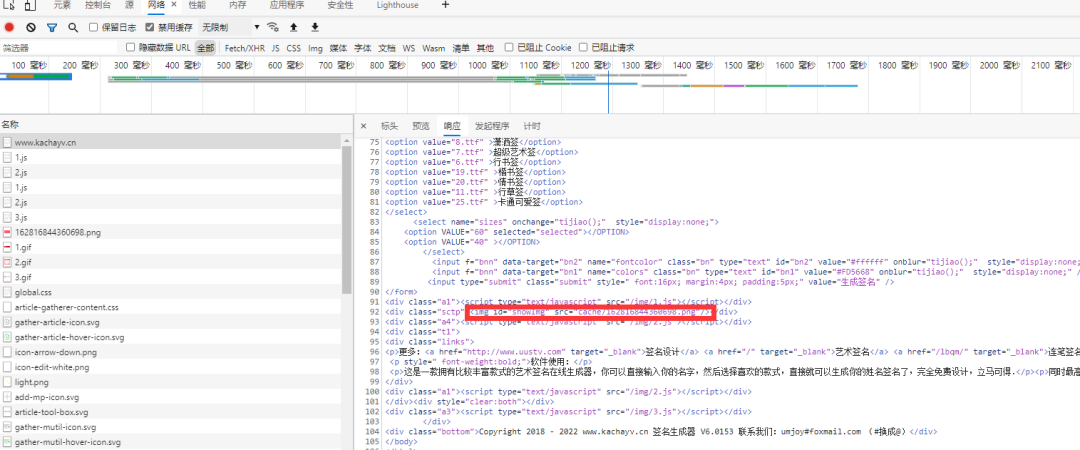`<img id="showImg" src="cache/162816844360698.png"/> `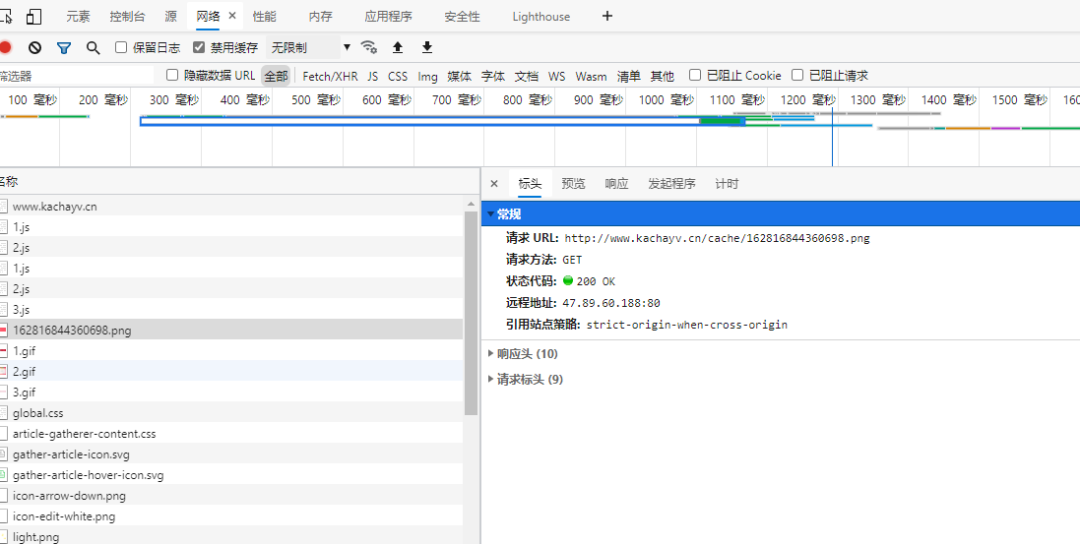• 将姓名、字体参数发送请求，获取到一个 HTML；
• 解析 HTML，找到签名图片名称；
• 发送获取图片请求，得到签名图片。

# 请求签名图片

`word: 姓名 fonts: 字体 sizes: 字体大小 fontcolor: 字体颜色 colors: 签名背景颜色`

```url = 'http://www.kachayv.cn/' data = { 'word': name, 'fonts': mapping_list[font], 'sizes': 60, 'fontcolor': '#ffffff', 'colors': '#FD5668' }
result = requests.post(url, data=data)
result.encoding = 'utf-8' html = result.text
print(html)
p = re.compile('<img id="showImg" src="cache/(.*?)"/>')
match = p.findall(html)
urlretrieve('http://www.kachayv.cn/cache/' + match, './pic.jpg')```

# 设计和实现软件界面

`def draw_window(self): self.init_window = Tk() self.init_window.title("阿花专属签名设计") self.init_window.geometry("800x500") self.init_window.geometry("+400+200") # 姓名 self.name_label = Label(self.init_window, text='鼎鼎大名', font=('微软雅黑', 16), fg='black') self.name_label.grid(row=0, column=0, columnspan=1) self.name_entry = Entry(self.init_window, font=('宋体', 16)) self.name_entry.grid(row=0, column=1) # 选择字体模式 self.font_label = Label(self.init_window, text='字体', font=('微软雅黑', 16), fg='black') self.font_label.grid(row=0, column=5, columnspan=1) self.combox_list = ttk.Combobox(self.init_window, textvariable=StringVar()) self.combox_list.grid(row=0, column=6, sticky='W') self.combox_list["value"] = ("行书签", "超级艺术签", "潇洒签", "手写连笔字", "行草签", "花式签", "温柔女生", "个性签", "商务签", "正楷体", "楷书签", "情书签", "卡通可爱签") self.combox_list.current(0) # 选择第一个 # 触发按钮 self.button = Button(self.init_window, text='美好来袭', font=('微软雅黑', 16), command=self.get_sign) self.button.grid(row=1, column=3, rowspan=2, sticky='W') # 图片展示 self.pic_label = Label(self.init_window) self.pic_label.grid(row=3, column=1, rowspan=10, columnspan=5, sticky='NW')`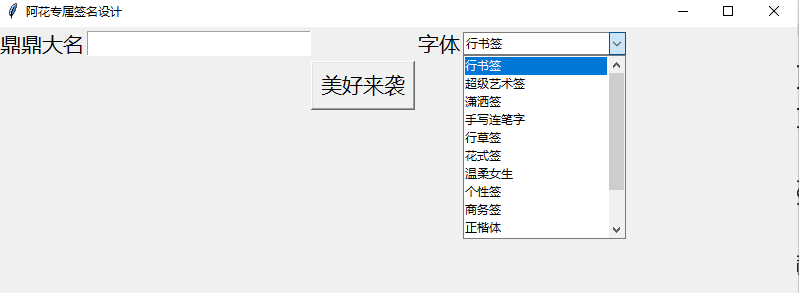# 合体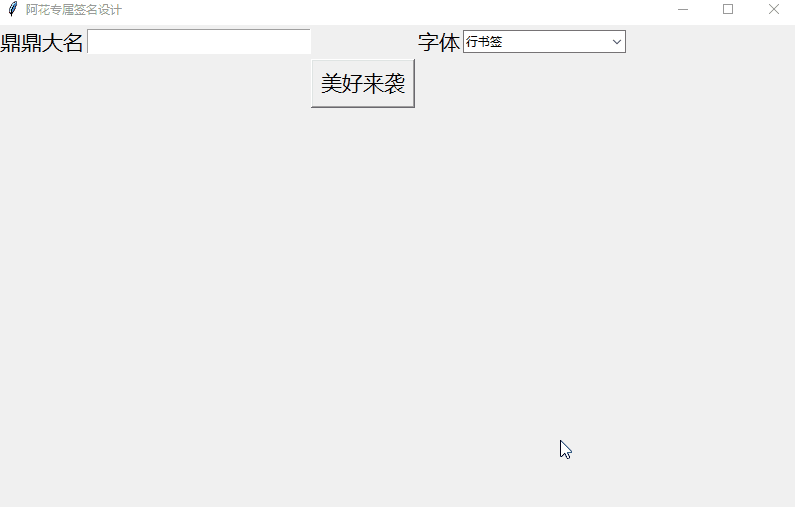OK